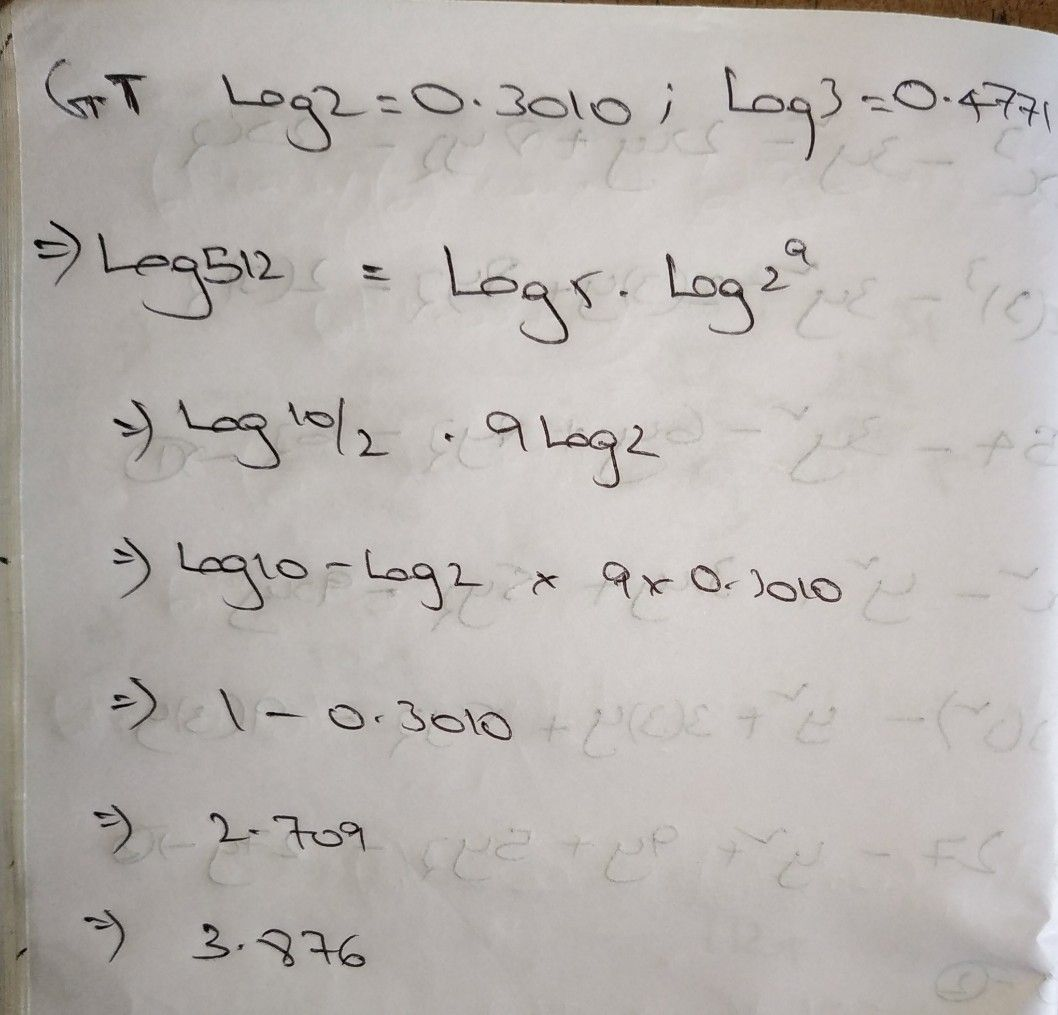Symbol
Problem$11411og2=0.3010$ and $0g3=0.47/1,$ the value of logs 512 i $A115\right)$ $\left(14$ $1.016$ $r^{1}$ $111110n$ $1og3312=$ $1o$ $β512$ $l00y3$ $loy^{7^{y}}$ $1o5\left(102\right)$ $910g2$ log $10-1082$ (9 $x0.3010\right)$ $1-0.3010$ $2.709$ $0.699$ $2709$ 699 $3.370$
Other
SolutionQanda teacher - kamesh